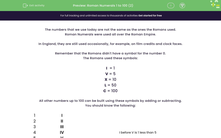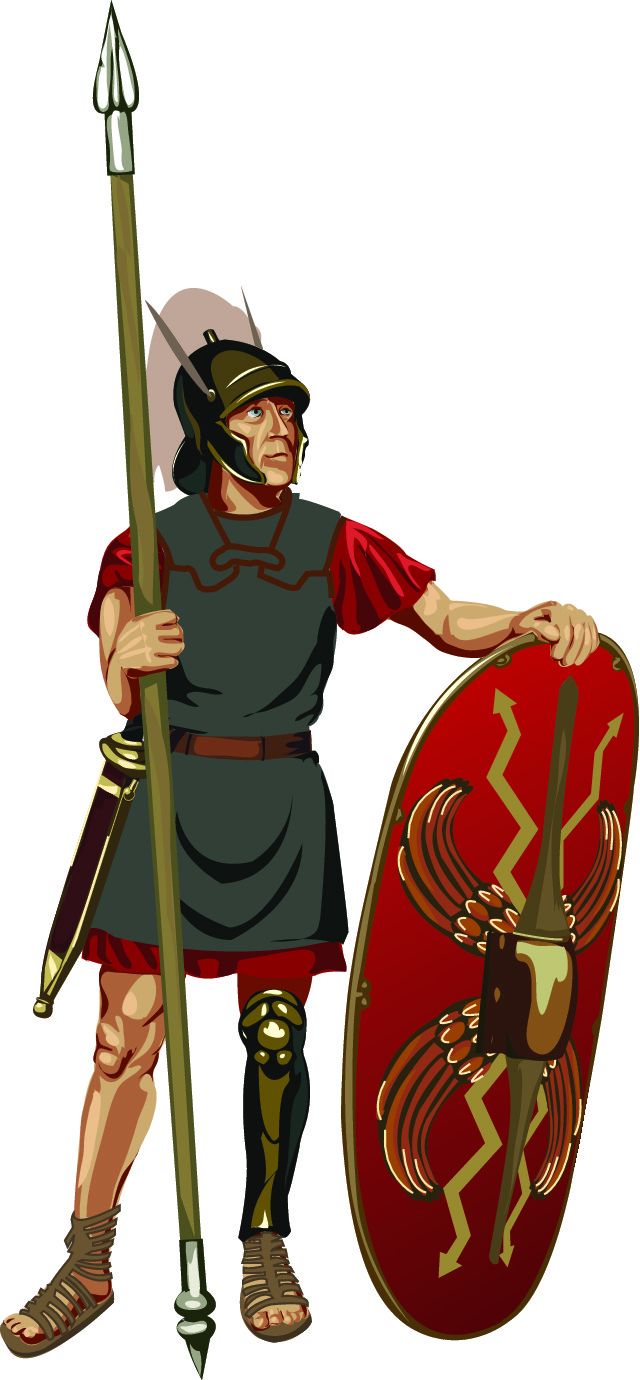# Convert Numbers up to 100 to Roman Numerals

In this worksheet, students will convert normal numbers to Roman numerals up to 100.Key stage:  KS 2

Curriculum topic:   Number: Number and Place Value

Curriculum subtopic:   Read Roman Numerals (I to C/1 to 100)

Difficulty level:#### Worksheet Overview

The numbers that we use today are not the same as the ones the Romans used.

Roman numerals were used all over the Roman Empire.In the United Kingdom, they are still used occasionally, for example, on film credits and clock faces.

Remember that the Romans didn't have a symbol for the number 0.

The Romans used these symbols:

I  = 1

V = 5

X = 10

L = 50

C = 100

All other numbers up to 100 can be built using these symbols by adding or subtracting.

You should know the following:

 1 I 2 II 3 III 4 IV I before V is 1 less than 5 5 V 6 VI I after V is 1 more than 5 7 VII II after V is 2 more than 5 8 VIII III after V is 3 more than 5 9 IX I before X is 1 less than 10 10 X 11 XI I after X is 1 more than 10 12 XII II after X is 2 more than 10

We build bigger numbers by adding 10s or Xs in Roman numerals.

 20 XX = X + X = 20 21 XXI = XX + I = 20 + 1 30 XXX = X + X + X = 30 37 XXXVII = XXX + VII = 30 + 7 40 XL = X before L is 10 less than 50 or 50 - 10 = 40 48 XLVIII = XL + VIII = 40 + 8 = 48 49 XLIX = XL + IX = 40 + 9 = 49 50 L 54 LIV = L + IV = 50 + 4 = 54 60 LX = X after L is 10 more than 50 or 50 + 10 = 60 67 LXVII = LX + VII = 60 + 7 = 67 70 LXX = L + XX = 50 + 20 = 70 80 LXXX = L + XXX = 50 + 30 = 80 84 LXXIV = L + XXXIV = 50 + 34 = 84 90 XC = X before C is 10 less than 100 or 100 - 10 = 90 96 XCVI = XC + VI = 90 + 6 = 96

Example

Convert 89 and 97 to Roman numerals.

89 = 50 + 39 = L + XXXIX = LXXXIX

97 = 90 + 7 = XC + VII = XCVII

Let's have a go at some questions now.

Remember that you can check back to this page at any point by clicking on the red help button on the screen.### What is EdPlace?

We're your National Curriculum aligned online education content provider helping each child succeed in English, maths and science from year 1 to GCSE. With an EdPlace account you’ll be able to track and measure progress, helping each child achieve their best. We build confidence and attainment by personalising each child’s learning at a level that suits them.

Get started